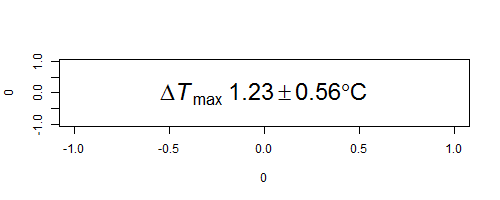# R plotmath functions combined with variable values

Getting certain special symbols into R plots, combined with values that are currently stored in variables, has been an ongoing headache of mine. In particular, plotmath symbols such as the plus-minus sign (±), for which the plotmath command is `%+-%`, had always caused problems due to my inability to parse the R help documentation. For an example, I was trying to get the following simple value (a mean ± 1 SE for a temperature difference) inserted into a plot (a minimalist example):It turns out that the plotmath operators that are surrounded by percent signs need to be surrounded by empty quote marks to get them to appear correctly inside a `bquote()` call. To make the plot above, I used the following commands:

[code lang=”R”]
# Define some variables
mymean = 1.2345678
mySE = 0.55555
# Make the empty plot
plot(x = 0, y = 0, type = ‘n’)
# Assemble a label, using bquote()
mylabel = bquote(Delta*italic(T)[max]~.(format(mymean,digits=3))*”%+-%”*
.(format(mySE,digits=2))*degree*C)
# Add mylabel to the plot at x=0, y=0
text(x = 0, y = 0, labels = mylabel, font = 2, cex = 2)
[/code]

The `bquote()` is where the action happens. Inside `bquote()`, I have the following arguments:

• `Delta` gets interpreted as a keyword, and turned in to the greek symbol.
• The asterisk makes sure Delta is directly next to whatever follows the asterisk (instead of the ~ tilde symbol, which inserts a blank space).
• `italic(T)` makes the capital letter T appear in italics.
• `[max]` makes whatever is inside the brackets into a subscript. A superscript would be set using the ^ symbol.
• The tilde puts a space after the subscript
• `.(format(mymean,dig=3))` evaluates the variable inside `.()` and returns the value. In this case, I have inserted a call to `format()` to make sure the value of `mymean` is displayed with a sensible number of significant digits.
• The asterisk leads into the `''%+-%''` chunk. The empty quotes around `%+-%` allow it to work here and be converted into the ± symbol.
• The next asterisk leads into `.(format(mySE,digits=2))`, which is another call to `.()` used to retrieve and print the numeric value stored in `mySE`, with the appropriate number of digits.
• The next asterisk leads into `degree`, which is another keyword, and R will convert it into the degree symbol °.
• Finally, the next asterisk leads to `C`, which just gets interpreted as the letter C, since it is not a keyword. I could have written a whole word here if desired (Celsius), or even multiple words separated by ~ tildes.

Once `mylabel` is assembled, I stick it in the plot at a location defined in the `text()` function.

The complete list of keywords and operator symbols available for use in this way can be found by typing `?plotmath` at the R console and perusing the help file.